# Mean State

Period Mean (original grids) [Watt m-2]
Model Period Mean (intersection) [Watt m-2]
Model Period Mean (complement) [Watt m-2]
Benchmark Period Mean (intersection) [Watt m-2]
Benchmark Period Mean (complement) [Watt m-2]
Bias [Watt m-2]
RMSE [Watt m-2]
Phase Shift [months]
Bias Score 
RMSE Score 
Seasonal Cycle Score 
Spatial Distribution Score 
Interannual Variability Score 
Overall Score 
Benchmark [-] -56.8
CRUNCEPv7 [-] -54.2 -54.0 -57.0 -52.3 3.39 13.3 2.44 0.612 0.464 0.614 0.994 0.537 0.614
GSWP3v1 [-] -64.0 -63.8 -57.0 -52.3 -7.03 15.1 1.71 0.485 0.462 0.756 0.951 0.816 0.655
WFDEI [-] -43.4 -42.9 -57.0 -52.3 14.0 20.1 2.96 0.347 0.431 0.515 0.965 0.724 0.569
Period Mean (original grids) [Watt m-2]
Model Period Mean (intersection) [Watt m-2]
Model Period Mean (complement) [Watt m-2]
Benchmark Period Mean (intersection) [Watt m-2]
Benchmark Period Mean (complement) [Watt m-2]
Bias [Watt m-2]
RMSE [Watt m-2]
Phase Shift [months]
Bias Score 
RMSE Score 
Seasonal Cycle Score 
Spatial Distribution Score 
Interannual Variability Score 
Overall Score 
Benchmark [-] -87.0
CRUNCEPv7 [-] -84.2 -85.0 -87.4 -58.4 2.88 17.7 0.342 0.809 0.598 0.969 0.963 0.520 0.743
GSWP3v1 [-] -91.7 -92.4 -87.4 -58.4 -4.61 18.6 0.227 0.764 0.603 0.977 0.899 0.716 0.760
WFDEI [-] -73.9 -74.8 -87.4 -58.4 12.8 22.0 0.651 0.663 0.580 0.925 0.996 0.720 0.744
Period Mean (original grids) [Watt m-2]
Model Period Mean (intersection) [Watt m-2]
Model Period Mean (complement) [Watt m-2]
Benchmark Period Mean (intersection) [Watt m-2]
Benchmark Period Mean (complement) [Watt m-2]
Bias [Watt m-2]
RMSE [Watt m-2]
Phase Shift [months]
Bias Score 
RMSE Score 
Seasonal Cycle Score 
Spatial Distribution Score 
Interannual Variability Score 
Overall Score 
Benchmark [-] -81.4
CRUNCEPv7 [-] -77.5 -77.7 -82.3 -62.6 6.42 19.0 1.28 0.682 0.499 0.829 0.976 0.482 0.661
GSWP3v1 [-] -86.5 -86.6 -82.3 -62.6 -2.65 16.8 0.707 0.708 0.529 0.918 0.960 0.711 0.726
WFDEI [-] -76.7 -76.9 -82.3 -62.6 6.70 19.4 1.14 0.662 0.489 0.846 0.996 0.623 0.684
Period Mean (original grids) [Watt m-2]
Model Period Mean (intersection) [Watt m-2]
Model Period Mean (complement) [Watt m-2]
Benchmark Period Mean (intersection) [Watt m-2]
Benchmark Period Mean (complement) [Watt m-2]
Bias [Watt m-2]
RMSE [Watt m-2]
Phase Shift [months]
Bias Score 
RMSE Score 
Seasonal Cycle Score 
Spatial Distribution Score 
Interannual Variability Score 
Overall Score 
Benchmark [-] -37.2
CRUNCEPv7 [-] -59.2 -59.2 -36.9 -41.0 -22.2 24.9 1.05 0.257 0.510 0.876 0.788 0.509 0.575
GSWP3v1 [-] -39.1 -39.1 -36.9 -41.0 -2.99 15.8 1.18 0.729 0.414 0.846 0.973 0.877 0.709
WFDEI [-] -55.0 -55.1 -36.9 -41.0 -18.4 21.5 1.14 0.327 0.510 0.858 0.746 0.527 0.580
Period Mean (original grids) [Watt m-2]
Model Period Mean (intersection) [Watt m-2]
Model Period Mean (complement) [Watt m-2]
Benchmark Period Mean (intersection) [Watt m-2]
Benchmark Period Mean (complement) [Watt m-2]
Bias [Watt m-2]
RMSE [Watt m-2]
Phase Shift [months]
Bias Score 
RMSE Score 
Seasonal Cycle Score 
Spatial Distribution Score 
Interannual Variability Score 
Overall Score 
Benchmark [-] -44.6
CRUNCEPv7 [-] -27.8 -27.7 -43.2 -49.2 16.1 18.5 3.17 0.0963 0.364 0.461 0.844 0.510 0.440
GSWP3v1 [-] -47.9 -47.8 -43.2 -49.2 -4.20 9.59 1.92 0.384 0.350 0.702 0.982 0.783 0.592
WFDEI [-] -21.3 -21.2 -43.2 -49.2 21.5 22.9 2.56 0.0570 0.291 0.593 0.902 0.806 0.490
Period Mean (original grids) [Watt m-2]
Model Period Mean (intersection) [Watt m-2]
Model Period Mean (complement) [Watt m-2]
Benchmark Period Mean (intersection) [Watt m-2]
Benchmark Period Mean (complement) [Watt m-2]
Bias [Watt m-2]
RMSE [Watt m-2]
Phase Shift [months]
Bias Score 
RMSE Score 
Seasonal Cycle Score 
Spatial Distribution Score 
Interannual Variability Score 
Overall Score 
Benchmark [-] -42.1
CRUNCEPv7 [-] -65.9 -65.9 -41.9 -51.7 -23.9 26.9 0.974 0.229 0.472 0.893 0.743 0.529 0.556
GSWP3v1 [-] -51.6 -51.6 -41.9 -51.7 -9.58 15.4 0.649 0.556 0.487 0.924 0.984 0.892 0.722
WFDEI [-] -62.8 -62.8 -41.9 -51.7 -20.9 24.5 0.925 0.276 0.459 0.901 0.686 0.533 0.552
Period Mean (original grids) [Watt m-2]
Model Period Mean (intersection) [Watt m-2]
Model Period Mean (complement) [Watt m-2]
Benchmark Period Mean (intersection) [Watt m-2]
Benchmark Period Mean (complement) [Watt m-2]
Bias [Watt m-2]
RMSE [Watt m-2]
Phase Shift [months]
Bias Score 
RMSE Score 
Seasonal Cycle Score 
Spatial Distribution Score 
Interannual Variability Score 
Overall Score 
Benchmark [-] -67.1
CRUNCEPv7 [-] -82.5 -82.3 -67.3 -59.4 -14.0 22.3 1.24 0.481 0.469 0.825 0.937 0.523 0.617
GSWP3v1 [-] -73.4 -73.1 -67.3 -59.4 -5.00 16.4 0.937 0.666 0.503 0.868 0.995 0.802 0.723
WFDEI [-] -79.1 -79.0 -67.3 -59.4 -10.8 21.2 1.29 0.553 0.433 0.815 0.982 0.550 0.628
Period Mean (original grids) [Watt m-2]
Model Period Mean (intersection) [Watt m-2]
Model Period Mean (complement) [Watt m-2]
Benchmark Period Mean (intersection) [Watt m-2]
Benchmark Period Mean (complement) [Watt m-2]
Bias [Watt m-2]
RMSE [Watt m-2]
Phase Shift [months]
Bias Score 
RMSE Score 
Seasonal Cycle Score 
Spatial Distribution Score 
Interannual Variability Score 
Overall Score 
Benchmark [-] -71.2
CRUNCEPv7 [-] -68.9 -68.9 -72.6 -53.4 7.97 20.9 1.14 0.620 0.521 0.858 0.987 0.479 0.664
GSWP3v1 [-] -77.5 -77.7 -72.6 -53.4 -2.50 17.1 0.848 0.709 0.556 0.893 0.957 0.704 0.729
WFDEI [-] -61.0 -61.0 -72.6 -53.4 14.9 24.5 1.24 0.489 0.500 0.832 0.987 0.631 0.656
Period Mean (original grids) [Watt m-2]
Model Period Mean (intersection) [Watt m-2]
Model Period Mean (complement) [Watt m-2]
Benchmark Period Mean (intersection) [Watt m-2]
Benchmark Period Mean (complement) [Watt m-2]
Bias [Watt m-2]
RMSE [Watt m-2]
Phase Shift [months]
Bias Score 
RMSE Score 
Seasonal Cycle Score 
Spatial Distribution Score 
Interannual Variability Score 
Overall Score 
Benchmark [-] -31.1
CRUNCEPv7 [-] -56.5 -56.6 -31.1 -32.1 -25.4 27.3 0.813 0.203 0.533 0.928 0.939 0.529 0.611
GSWP3v1 [-] -38.2 -38.3 -31.1 -32.1 -7.23 15.2 0.805 0.630 0.449 0.919 0.952 0.896 0.716
WFDEI [-] -54.1 -54.2 -31.1 -32.1 -23.1 25.6 1.19 0.238 0.494 0.861 0.895 0.549 0.589
Period Mean (original grids) [Watt m-2]
Model Period Mean (intersection) [Watt m-2]
Model Period Mean (complement) [Watt m-2]
Benchmark Period Mean (intersection) [Watt m-2]
Benchmark Period Mean (complement) [Watt m-2]
Bias [Watt m-2]
RMSE [Watt m-2]
Phase Shift [months]
Bias Score 
RMSE Score 
Seasonal Cycle Score 
Spatial Distribution Score 
Interannual Variability Score 
Overall Score 
Benchmark [-] -96.8
CRUNCEPv7 [-] -93.0 -93.0 -99.4 -63.9 9.20 20.9 0.539 0.558 0.508 0.922 0.841 0.483 0.637
GSWP3v1 [-] -96.0 -96.1 -99.4 -63.9 6.20 16.5 0.483 0.666 0.542 0.936 0.921 0.733 0.723
WFDEI [-] -92.3 -92.4 -99.4 -63.9 9.55 22.1 0.465 0.559 0.461 0.936 0.838 0.650 0.651
Period Mean (original grids) [Watt m-2]
Model Period Mean (intersection) [Watt m-2]
Model Period Mean (complement) [Watt m-2]
Benchmark Period Mean (intersection) [Watt m-2]
Benchmark Period Mean (complement) [Watt m-2]
Bias [Watt m-2]
RMSE [Watt m-2]
Phase Shift [months]
Bias Score 
RMSE Score 
Seasonal Cycle Score 
Spatial Distribution Score 
Interannual Variability Score 
Overall Score 
Benchmark [-] -55.6
CRUNCEPv7 [-] -70.5 -70.5 -55.1 -60.8 -14.9 19.6 0.976 0.337 0.465 0.878 0.526 0.519 0.532
GSWP3v1 [-] -57.8 -57.8 -55.1 -60.8 -3.30 11.5 0.743 0.663 0.471 0.910 0.964 0.869 0.725
WFDEI [-] -66.3 -66.3 -55.1 -60.8 -11.5 17.4 0.834 0.409 0.436 0.902 0.609 0.547 0.556
Period Mean (original grids) [Watt m-2]
Model Period Mean (intersection) [Watt m-2]
Model Period Mean (complement) [Watt m-2]
Benchmark Period Mean (intersection) [Watt m-2]
Benchmark Period Mean (complement) [Watt m-2]
Bias [Watt m-2]
RMSE [Watt m-2]
Phase Shift [months]
Bias Score 
RMSE Score 
Seasonal Cycle Score 
Spatial Distribution Score 
Interannual Variability Score 
Overall Score 
Benchmark [-] -52.6
CRUNCEPv7 [-] -70.6 -70.6 -65.2 -46.5 -3.71 22.1 1.25 0.449 0.479 0.826 0.898 0.488 0.603
GSWP3v1 [-] -70.0 -70.0 -65.2 -46.5 -3.58 16.7 0.949 0.594 0.496 0.869 0.932 0.767 0.692
WFDEI [-] -68.2 -68.2 -65.2 -46.5 -1.70 22.4 1.26 0.442 0.457 0.825 0.900 0.593 0.612
Period Mean (original grids) [Watt m-2]
Model Period Mean (intersection) [Watt m-2]
Model Period Mean (complement) [Watt m-2]
Benchmark Period Mean (intersection) [Watt m-2]
Benchmark Period Mean (complement) [Watt m-2]
Bias [Watt m-2]
RMSE [Watt m-2]
Phase Shift [months]
Bias Score 
RMSE Score 
Seasonal Cycle Score 
Spatial Distribution Score 
Interannual Variability Score 
Overall Score 
Benchmark [-] -121.
CRUNCEPv7 [-] -104. -104. -122. -81.3 20.0 32.1 0.849 0.503 0.470 0.887 0.802 0.424 0.593
GSWP3v1 [-] -108. -108. -122. -81.3 15.3 25.8 0.675 0.563 0.530 0.911 0.818 0.633 0.664
WFDEI [-] -107. -107. -122. -81.3 16.2 29.3 0.839 0.553 0.468 0.895 0.749 0.490 0.604
Period Mean (original grids) [Watt m-2]
Model Period Mean (intersection) [Watt m-2]
Model Period Mean (complement) [Watt m-2]
Benchmark Period Mean (intersection) [Watt m-2]
Benchmark Period Mean (complement) [Watt m-2]
Bias [Watt m-2]
RMSE [Watt m-2]
Phase Shift [months]
Bias Score 
RMSE Score 
Seasonal Cycle Score 
Spatial Distribution Score 
Interannual Variability Score 
Overall Score 
Benchmark [-] -34.3
CRUNCEPv7 [-] -60.2 -60.2 -34.2 -36.5 -25.5 27.3 0.658 0.188 0.523 0.928 0.950 0.499 0.602
GSWP3v1 [-] -42.3 -42.3 -34.2 -36.5 -8.62 15.2 0.830 0.578 0.458 0.891 0.822 0.883 0.682
WFDEI [-] -57.5 -57.5 -34.2 -36.5 -23.1 25.4 0.649 0.220 0.497 0.932 0.986 0.528 0.610
Period Mean (original grids) [Watt m-2]
Model Period Mean (intersection) [Watt m-2]
Model Period Mean (complement) [Watt m-2]
Benchmark Period Mean (intersection) [Watt m-2]
Benchmark Period Mean (complement) [Watt m-2]
Bias [Watt m-2]
RMSE [Watt m-2]
Phase Shift [months]
Bias Score 
RMSE Score 
Seasonal Cycle Score 
Spatial Distribution Score 
Interannual Variability Score 
Overall Score 
Benchmark [-] -70.7
CRUNCEPv7 [-] -69.5 -69.4 -73.4 -57.5 9.50 17.8 1.36 0.493 0.476 0.790 0.976 0.515 0.621
GSWP3v1 [-] -81.0 -80.9 -73.4 -57.5 -4.62 14.4 0.925 0.583 0.495 0.859 0.994 0.793 0.703
WFDEI [-] -68.7 -68.7 -73.4 -57.5 9.08 19.8 1.63 0.469 0.436 0.741 0.953 0.698 0.622
Period Mean (original grids) [Watt m-2]
Model Period Mean (intersection) [Watt m-2]
Model Period Mean (complement) [Watt m-2]
Benchmark Period Mean (intersection) [Watt m-2]
Benchmark Period Mean (complement) [Watt m-2]
Bias [Watt m-2]
RMSE [Watt m-2]
Phase Shift [months]
Bias Score 
RMSE Score 
Seasonal Cycle Score 
Spatial Distribution Score 
Interannual Variability Score 
Overall Score 
Benchmark [-] -53.2
CRUNCEPv7 [-] -66.3 -66.2 -52.9 -58.5 -12.3 21.3 3.99 0.379 0.287 0.329 0.938 0.620 0.473
GSWP3v1 [-] -60.7 -60.5 -52.9 -58.5 -7.65 12.0 0.781 0.531 0.478 0.876 0.998 0.892 0.709
WFDEI [-] -65.0 -64.8 -52.9 -58.5 -11.3 19.6 3.21 0.415 0.300 0.471 0.996 0.780 0.544
Period Mean (original grids) [Watt m-2]
Model Period Mean (intersection) [Watt m-2]
Model Period Mean (complement) [Watt m-2]
Benchmark Period Mean (intersection) [Watt m-2]
Benchmark Period Mean (complement) [Watt m-2]
Bias [Watt m-2]
RMSE [Watt m-2]
Phase Shift [months]
Bias Score 
RMSE Score 
Seasonal Cycle Score 
Spatial Distribution Score 
Interannual Variability Score 
Overall Score 
Benchmark [-] -42.5
CRUNCEPv7 [-] -69.0 -69.0 -42.4 -45.4 -26.5 29.2 0.853 0.209 0.486 0.890 0.727 0.517 0.553
GSWP3v1 [-] -53.7 -53.7 -42.4 -45.4 -11.0 15.8 0.545 0.526 0.514 0.937 0.988 0.863 0.724
WFDEI [-] -66.6 -66.6 -42.4 -45.4 -24.2 27.1 0.683 0.238 0.492 0.921 0.790 0.557 0.582
Period Mean (original grids) [Watt m-2]
Model Period Mean (intersection) [Watt m-2]
Model Period Mean (complement) [Watt m-2]
Benchmark Period Mean (intersection) [Watt m-2]
Benchmark Period Mean (complement) [Watt m-2]
Bias [Watt m-2]
RMSE [Watt m-2]
Phase Shift [months]
Bias Score 
RMSE Score 
Seasonal Cycle Score 
Spatial Distribution Score 
Interannual Variability Score 
Overall Score 
Benchmark [-] -80.3
CRUNCEPv7 [-] -89.5 -89.2 -80.8 -54.9 -8.00 21.9 0.511 0.633 0.519 0.935 0.661 0.498 0.628
GSWP3v1 [-] -85.7 -85.5 -80.8 -54.9 -4.42 18.0 0.271 0.727 0.565 0.969 0.931 0.762 0.753
WFDEI [-] -89.1 -88.7 -80.8 -54.9 -7.63 21.7 0.723 0.663 0.500 0.896 0.766 0.585 0.652
Period Mean (original grids) [Watt m-2]
Model Period Mean (intersection) [Watt m-2]
Model Period Mean (complement) [Watt m-2]
Benchmark Period Mean (intersection) [Watt m-2]
Benchmark Period Mean (complement) [Watt m-2]
Bias [Watt m-2]
RMSE [Watt m-2]
Phase Shift [months]
Bias Score 
RMSE Score 
Seasonal Cycle Score 
Spatial Distribution Score 
Interannual Variability Score 
Overall Score 
Benchmark [-] -43.7
CRUNCEPv7 [-] -31.5 -31.5 -43.6 -47.7 12.1 16.5 1.66 0.334 0.445 0.765 0.736 0.539 0.544
GSWP3v1 [-] -50.9 -50.8 -43.6 -47.7 -7.23 12.5 1.59 0.496 0.459 0.781 0.916 0.752 0.644
WFDEI [-] -24.9 -24.9 -43.6 -47.7 18.6 22.0 1.30 0.209 0.414 0.837 0.691 0.760 0.554
Period Mean (original grids) [Watt m-2]
Model Period Mean (intersection) [Watt m-2]
Model Period Mean (complement) [Watt m-2]
Benchmark Period Mean (intersection) [Watt m-2]
Benchmark Period Mean (complement) [Watt m-2]
Bias [Watt m-2]
RMSE [Watt m-2]
Phase Shift [months]
Bias Score 
RMSE Score 
Seasonal Cycle Score 
Spatial Distribution Score 
Interannual Variability Score 
Overall Score 
Benchmark [-] -64.5
CRUNCEPv7 [-] -61.3 -61.7 -64.8 -56.3 3.81 14.7 1.45 0.617 0.485 0.792 0.766 0.506 0.608
GSWP3v1 [-] -69.7 -70.1 -64.8 -56.3 -4.85 13.0 0.736 0.713 0.484 0.905 0.974 0.810 0.728
WFDEI [-] -57.6 -57.9 -64.8 -56.3 7.30 16.3 1.35 0.613 0.443 0.812 0.865 0.720 0.649
Period Mean (original grids) [Watt m-2]
Model Period Mean (intersection) [Watt m-2]
Model Period Mean (complement) [Watt m-2]
Benchmark Period Mean (intersection) [Watt m-2]
Benchmark Period Mean (complement) [Watt m-2]
Bias [Watt m-2]
RMSE [Watt m-2]
Phase Shift [months]
Bias Score 
RMSE Score 
Seasonal Cycle Score 
Spatial Distribution Score 
Interannual Variability Score 
Overall Score 
Benchmark [-] -80.0
CRUNCEPv7 [-] -94.0 -93.8 -83.0 -54.0 -8.53 24.7 1.09 0.421 0.448 0.851 0.609 0.466 0.540
GSWP3v1 [-] -86.3 -85.9 -83.0 -54.0 -0.276 20.0 0.784 0.527 0.441 0.888 0.889 0.768 0.659
WFDEI [-] -91.4 -91.4 -83.0 -54.0 -6.63 23.1 1.05 0.445 0.431 0.863 0.862 0.544 0.596

# Temporally integrated period mean

BENCHMARK MEAN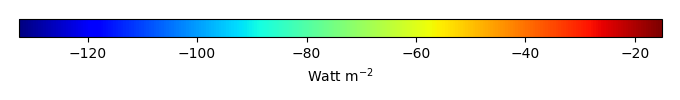MODEL MEANBIAS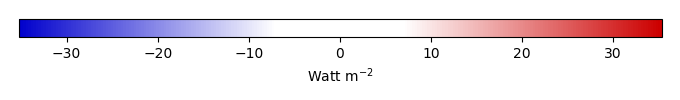BIAS SCORERMSE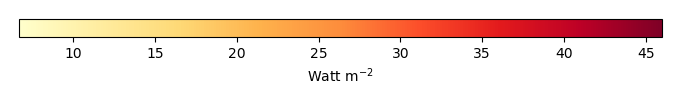RMSE SCOREBENCHMARK INTERANNUAL VARIABILITY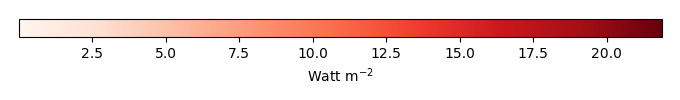MODEL INTERANNUAL VARIABILITYINTERANNUAL VARIABILITY SCOREBENCHMARK MAX MONTHMODEL MAX MONTHDIFFERENCE IN MAX MONTH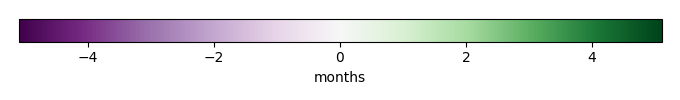SEASONAL CYCLE SCORESPATIAL TAYLOR DIAGRAMMODEL COLORS# Spatially integrated regional mean

MODEL COLORSREGIONAL MEANANNUAL CYCLEMONTHLY ANOMALYANNUAL CYCLE# All Models

BenchmarkCRUNCEPv7GSWP3v1WFDEI# Data Information

creation_date: Tue Jul 1 08:25:00 PDT 2014

source_file: This product is generated from monthly 1 degree GEWEX SRB Radiation observations